# Area and perimeter of 2D shapes

If you want to learn more about finding the area and perimeter of 2D shapes, you have come to the right place!

This blog includes diagrams of 2D shapes labelled with the associated area and perimeter vocabulary, as well as taking you through the steps for finding the area and perimeter of 2D shapes using geometry formulas. Don't forget you can download all the area and perimeter poster images included in this blog for free.

If you want to know more about 2D shapes, 2D shape angles or symmetry make sure you read our collections of maths blogs.

## What is the perimeter of a shape?

A shape's perimeter is the distance around the outside of a 2D shape. The perimeter of a circle is called the circumference.

## What is the area of a shape?

The area of a shape is the amount of space inside the lines or boundary of a 2D shape. The area of a shape can sometimes be found by counting squares inside the shape. The area of a shape can also be found using a mathematical formula.

## How do you find the perimeter of a rectangle?

The perimeter of a rectangle can be found by adding together the lengths of the sides. The formula to work out the perimeter of a rectangle is P = 2 ( l + w ) or perimeter equals the length plus the width, multiplied by two.

Don’t forget all squares are rectangles but not all rectangles are squares! Find out more about 2D shapes in our 2D shape blog.

See if you can find the perimeter of these two rectangles:Use P = 2 ( l + w ) to find the perimeter of a rectangle

Rectangle one is a square. It has four equal sides all measuring 6 cm.

P = 6 + 6 + 6 + 6

P = 24 cm

Or

P = 2 ( l + w )

P = 2 (6 + 6)

P = 2 (12)

P = 2 x 12

P = 24 cm

Rectangle two is not a square. It has two sets of equal sides. One set measures 5 cm each the other set measures 12 cm each.

P = 5 + 12 + 5 + 12

P = 34 cm

Or

P = 2 ( l + w )

P = 2 (5 + 12)

P = 2 (17)

P = 34 cm

## How do you find the area of a rectangle?

The area of a rectangle can be found by counting the squares inside its boundary lines or using a formula.

See if you can find the area of these two rectangles by counting the squares:Use A = wl to find the area of a rectangle

Did you work out the areas of the rectangles correctly?

Rectangle three has an area of 9 cm2

Rectangle four has an area of 45 m2

The area of a rectangle can also be worked out using the formula A = wl or area equals width multiplied by length.

Use this formula to find the area of these two rectangles: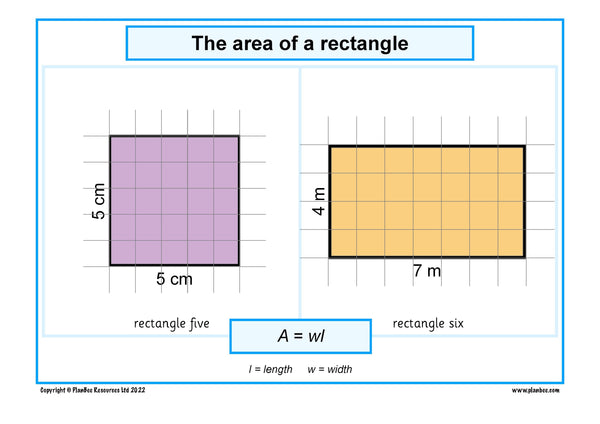The area of a rectangle A = wl

Rectangle five is a square. The width and the length of the sides are both 5 cm.

A = wl

A = 5 x 5

A = 25 cm2

Rectangle six is not a square. The width of the sides are 4 m. The length of the sides are 7 m.

A = wl

A = 4 x 7

A = 24 m2

## What is a rectilinear shape?

A rectilinear shape is a shape with straight sides and right angles. It can look like multiple rectangles that have been joined together.

If you want to know more about 2D shapes make sure you read our shape blogs!

## How do you find the perimeter of a rectilinear shape?

You can find the perimeter of a rectilinear shape by adding together the length of each of the sides.

See if you can find the perimeter of these two rectilinear shapes:Find the perimeter of these rectilinear shapes

Rectilinear shape one has eight sides.

P = a + b + c + d + e + f + g + h

P = 3 + 1 + 1 + 3 + 1 + 3 + 1 + 1

P = 14 cm

Rectilinear shape two has six sides.

P = a + b + c + d + e + f

P = 2 + 6 + 8 + 2 + e + f

Oh dear! The measurements on the shape are only recorded for four sides.

Using the information we have it is possible to work out the length of side e and side f:

e = 8 - 2

e = 6 cm

f = 6 - 2

f = 4 cm

Now we can input the values of e and f into the formula to work out the perimeter of rectilinear shape two:

P = a + b + c + d + e + f

P = 2 + 6 + 8 + 2 + 6 + 4

P = 28 cm

## How do you find the area of a rectilinear shape?

The area of a rectilinear shape can be found by counting the squares inside its boundary lines or using a formula.

See if you can find the area of these two rectilinear shapes by counting the squares:Find the area of these rectilinear shapes

Rectilinear shape three has an area of 6 cm2

Rectilinear shape four has an area of 12 cm2

The area of a rectilinear shape can also be worked out by splitting the shape into rectangles and using the formula A = wl or area equals width multiplied by length to find the area of each rectangle before adding the separate areas together.

Use this formula to find the area of these two rectilinear shapes.Find the area of these rectilinear shapes

Rectilinear shape five can be separated into rectangular shapes in lots of different ways. For this example, we are going to separate it into four rectangles, one on each row of the grid.

Section 1

A = wl

A = 1 x 1

A = 1 cm2

Section 2

A = wl

A = 1 x 2

A = 2 cm2

Section 3

A = wl

A = 1 x 2

A = 2 cm2

Section 4

A = wl

A = 1 x 3

A = 3 cm2

Combining sections 1, 2, 3 and 4 will tell us the area of rectilinear shape five.

A = 1 + 2 + 2 + 3

A = 8 cm2

The area of rectilinear shape six can be worked out in different ways. For this example we are going to separate it into two shapes.

We can work out the area of the square, 4 cm x 4 cm, and then take away the missing chunk.

A = wl

A = 4 x 4

A = 16 cm2

Before we can subtract the missing rectangle we need to work out its area.

A = wl

A = 2 x 1

A = 2 cm2

Now subtract the area of the missing rectangle from the area of the bigger square:

A = 16 - 2

A = 14 cm2

## How do you find the perimeter of a triangle?

The perimeter of a triangle can be found by adding together the lengths of the sides. The formula to work out the perimeter of a rectangle is P = a + b + c.

See if you can find the perimeter of these two triangles: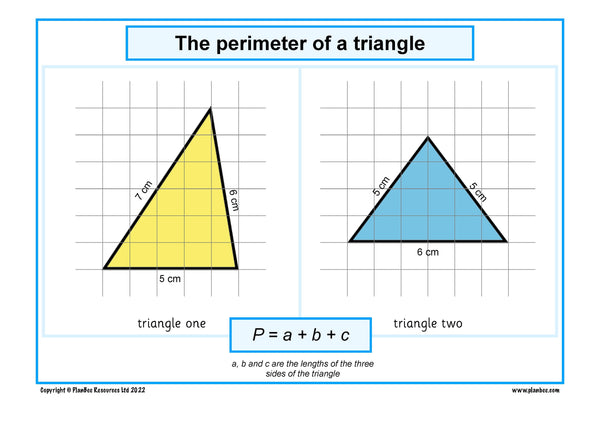Find the perimeter of these triangles using P = a + b + c

Triangle one has sides measuring 5 cm, 7 cm and 6 cm.

P = a + b + c

P = 5 + 7 + 6

P = 18 cm

Triangle two has sides measuring 6 cm, 5 cm and 5 cm.

P = a + b + c

P = 6 + 5 + 5

P = 16 cm

## How do you find the area of a triangle?

The area of a triangle can be found using the formula A = (hb b) ÷ 2. In this formula A is the area, hb is the height, b is the base. The height of the triangle is marked in the picture below with a dashed line.

Use this formula to find the area of these two triangles:Find the area of these triangles using the formula

Triangle three has a base (b) measuring 6 cm and height (hb) measuring 5 cm.

A = 15 cm2

Triangle four has a base measuring 5 cm and height measuring 5 cm.

A = 12.5 cm2

## How do you find the perimeter of a trapezium?

The perimeter of a trapezium can be found by adding together the lengths of the sides. The formula to work out the perimeter of a trapezium is P = a + b + c + d.

See if you can find the perimeter of these two trapeziums: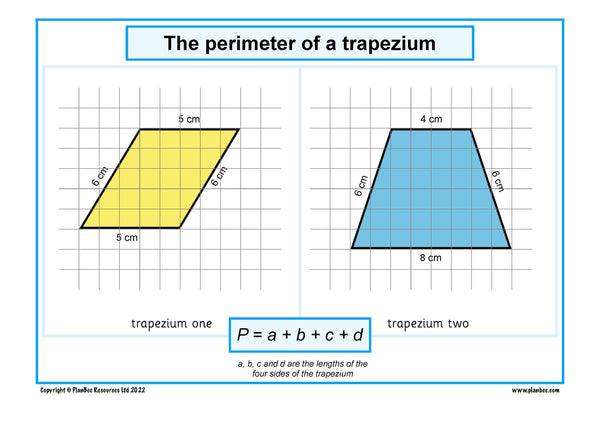Work out the perimeter of the trapeziums

Trapezium one has four sides measuring 5 cm, 6 cm, 5 cm and 6 cm.

P = a + b + c + d

P = 5 + 6 + 5 + 6

P = 22 cm

Trapezium two has four sides measuring 4 cm, 6 cm, 8 cm and 6 cm

P = a + b + c + d

P = 4 + 6 + 8 + 6

P = 24 cm

## How do you find the area of a trapezium?

The area of a trapezium can be found using the formula area equals half of a plus b divided by two and multiplied by h. a and b are the parallel lines. h is the height of the trapezium. It is marked in the picture below with a dashed line.

See if you can find the perimeter of these two trapeziums: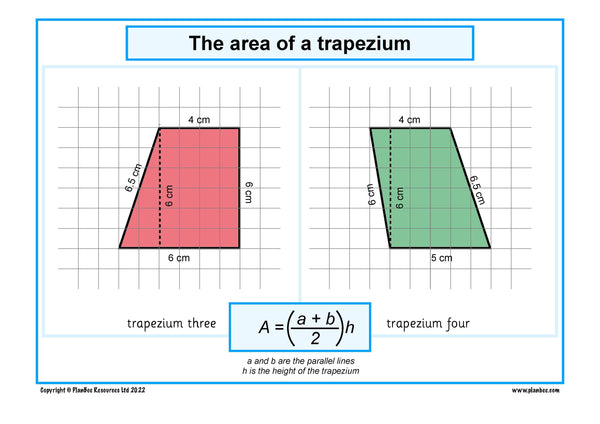Work out the area of the trapeziums

Trapezium three

a = 4 cm

b = 6 cm

h = 6 cm

A = 30 cm2

Trapezium four

a = 4 cm

b = 5 cm

h = 6 cm

A = 4.5 x 6

A = 27 cm2

## When are angles taught in the Primary Maths National Curriculum?

Area and perimeter are taught as part of the measurement strand of the Maths curriculum.

Perimeter is first taught in year 3 when children:

In year 4, children are introduced to area and build on their knowledge of perimeter when they:

Area and perimeter are taught in year 5 when children:

Area is taught in year 6 when children:

## Circles

If you want a challenge, have a sneak peek at how to find the area and perimeter of a circle. This content isn’t taught in the Maths National Curriculum until KS3.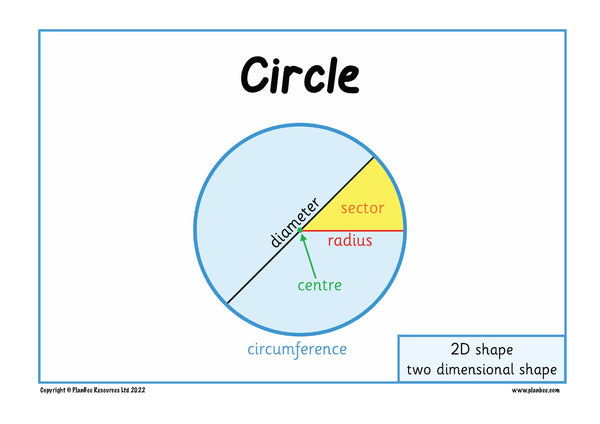The parts of a circle labelled

## How do you find the circumference of a circle?

The perimeter of a circle is called the circumference. It can be found using the formula C = 2 π r

In this formula C stands for circumference.

π is the mathematical symbol pi. π is a number approximately equal to 3.14

r represents the radius of the circle.

If you want to know more about squared numbers read our Square Numbers blog.

To find the circumference of a circle you need to know the radius of the circle. Remember the radius is half of the diameter of the circle. This means if you know the diameter of the circle you can half it to work out the radius by dividing the diameter by 2.Use the formula C = 2 π r to find the circumference of the circles

Work out the circumference of these two circles. One circle has a radius of 5cm. The other circle has a diameter of 8m. You can use a calculator to help you.

Circle one

r = 5 cm

C = 2 π r

C = 2 x 3.14 x 5

C = 31.4 cm

Circle two

If the diameter of the circle is 8m, the radius is 4m.

C = 2 π r

C = 2 x 3.14 x 4

C = 25.12 m

## How do you find the area of a circle?

The area of a circle can be found using the formula A = π r2

In this formula A stands for Area.

π is the mathematical symbol pi. π is a number approximately equal to 3.14

r represents the radius of the circle.

To find the area of a circle you need to know the radius of the circle. Remember the radius is half of the diameter of the circle. This means if you know the diameter of the circle you can half it to work out the radius by dividing the diameter by 2.

Work out the area of these two circles. One circle has a radius of 3cm. The other circle has a diameter of 4m. You can use a calculator to help you.Find the area of these circles

Circle three

r = 3 cm

A = π r2

A = 3.14 x 32

A = 3.14 x 9

A = 28.26 cm2

Circle four

d = 4 m

If d = 4m then r = 2m

A = π r2

A = 3.14 x 22

A = 3.14 x 4

A = 12.56 m2

The equation images in this blog have been powered by CodeCogs.